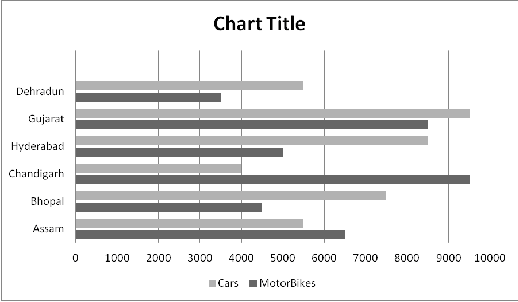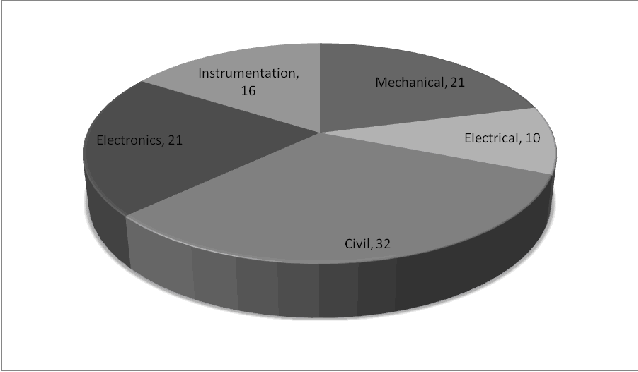# IBPS Quantitative Aptitude model test paper - 8

Home > Aptitude test > Quantitative model test - 8

## IBPS Quantitative Aptitude model test paper - 8

Q1. Study the following graph carefully to answer the questions that follow :

Total Sale of Bikes and Cars in Six different cities in an IndiaQ1. What is the difference between the total sale of Cars and Bikes in all the cities together ?

A. 6000
B. 5500
C. 3000
D. 1500

Q2. The sale of Bikes in Assam is approximately what percentage of the total sale of Bikes in all the cities together ?

A. 34%
B. 45%
C. 5%
D. 17%

Q3. What is the ratio of the sale of Cars in Chandigarh to the sale of Cars in Gujarat ?

A. 42%
B. 25%
C. 55%
D. 20%

Q4. The sale of Cars in cities Hyderabad and Bhopal together is approximately what percent of the sale of Cars in cities Gujarat, Assam and Dehradun together ?

A. 78%
B. 70%
C. 85%
D. 67%

Q5. What is the average sale of Cars in all the cities together ?

A. 6125
B. 6750
C. 6300
D. 6900

Directions (Q.6-10) In the following questions two equations numbered I and II are given. You have to solve both the equations and – Give answer if

A. a>b
B. a> = b
C. a< b
D. a=E. a= b or the relationship cannot be handled.

Q6. I. 2a2 = 3a
II. 8b2 = 26b -15.

(i) A (ii) B (iii) C (iv) D (v) E

Q7. I. √3
b2 + 11 b = -6√3

II. 3a2 – 14a + 8 = 0

(i) A (ii) B (iii) C (iv) D (v) E

Q8. I. √ (2a+9) = 13 –a
II. 7b2 – 5b – 2 = 0

(i) A (ii) B (iii) C (iv) D (v) E

Q9. I. a2 – 64 = 0.
II. b2 = 3136

(i) A (ii) B (iii) C (iv) D (v) E

Q10. I. 3a2 + 4a = 4.
II. b3 – 8 = 0

(i) A (ii) B (iii) C (iv) D (v) E

Directions (Q.11 - 13) In the following find the suitable value against (?) .

Q11. 1369 1521 1681 1849 ?

A. 1950
B. 2025
C. 2150
D. 1925
E. None of these

Q12. ? 42 48 54 60.

A. 32
B. 34
C. 24
D. 28
E. None of these

Q13. 15 16 ? 31 47

A. 19
B. 18
C. 28
D. 25
E. None of these

Directions (Q14-Q16). What will come in place of the question mark (?) in the following question.

Q14. 116 x 1212 ÷ 1771561 = (11) ?

A. 14
B. 3
C. 4
D. 6

Q15. 589 ÷ 13 x 15 – 159 = ? – 11.38 .

A. 532
B. 592
C. 621
D. 482

Q16. 39% of 485 – 22% of 516 = ? – 1008 .

A. 183.631
B. 281.63
C. 1016.63
D. 1083.63

Q17. Harish purchased a Jewellery marked at 536500 at a discount of 8%. If the VAT is charged at the rate of 10%, find the amount paid by the jewellery ?

A. 493580
B. 542938
C. 429200
D. None of these

Q18. In a school, the number of students two years ago was 6250. Due to admission to other colleges, the number of students decreases @8% every year. Find the decrease in the number of students of school in the last two years ?

A. 5290
B. 789
C. 960
D. 1040

Q19. Amit invests Rs. 9000 partly in 5%, Rs. 167 Shares @ Rs.240 and partly in 3% Rs. 700 shares @ Rs.850. If his total income is 500, how much has he invested in each ?

A. Rs. 5000 and Rs.4000
B. Rs. 3000 and Rs. 6000
C. Rs. 2000 and Rs. 7000
D. None of these

Q20. What will be the third proportional to 6 and 12 ?

A. 8
B. 15
C. 24
D. None of these

Directions (Q21-25) Study the pie-chart carefully to answer the questions that follow :

Percentage Wise Breakup of Employees in terms of Specialisation.Total Number of Employees are 16700.

Q21. What is the total number of employees having specialisation in Instrumentation, Electronics and Civil ?

A. 11523
B. 10521
C. 10980
D. 8851

Q22. Employees having Mechanical Engineering as specialisation approximately what percentage of employees having Civil as specialisation?

A. 21%
B. 152%
C. 65%
D. 35%

Q23. What is the total number of employees having Mechanical as specialisation?

A.5344
B.3507
C.3500
D. 4298

Q24. What is the ratio of the employees having Electrical as specialisation to employees having Electronics as specialisation?

A. 50%
B. 53%
C. 65%
D. 47%

Q25. Employees having Instrumentation forms approximately what percent of employees having Civil as specialisation ?

A. 48%
B. 50%
C.53%
D. 45%